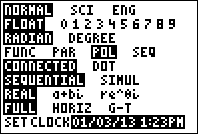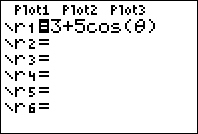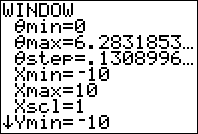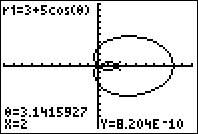# How to Graph Polar Equations on the TI-83+ and TI-84+

Filed under Algebra, Difficulty: Easy, TI-83 Plus, TI-84 Plus.

Polar coordinates are another way of representing a two-dimensional space, just like Cartesian (rectangular) coordinates. Instead of x and y, points are described using r, a radius, and θ (theta), the angle counterclockwise from the positive x axis.

In this tutorial, learn how to use your TI-83, TI-83 Plus, or TI-84 Plus to graph equations in polar variables. If you’re interested in a basic introduction to graphing features, check out our tutorial and video on graphing in rectangular coordinates.First, we need to make sure we have the correct settings selected. Check your mode menu by pressing the button. Use the arrow buttons to navigate, and to select an option. To enable polar graphing, make sure the “POL” or “POLAR” option is selected. Polar graphing behaves differently depending on whether you are in radian or degree mode—usually, you’ll want to work in radians.Mode menu on the TI-84+. “POL” is selected to enable polar graphing.

Next, we can go to the equation entry screen by pressing the button, just like for graphing equations in rectangular coordinates. This time, instead of seeing “y=” for each equation, it prompts for each equation in terms of r. If r is a constant, the result will be a circle; otherwise, r is a function of angle. Go ahead and enter the equation you want to graph.The equation entry screen with polar graphing on the TI-84+.

Before we graph our function, we must first confirm that the window sections are correct. Pressing the button lets us check these options. In addition to the traditional window settings, we are asked to specify θmin, θmax, and θstep. The default settings are 0, 2*π, and π/24, which means that the calculator will use 48 points to plot the function between θ=0 and θ=2*π. If you want your function to be smoother, you can decrease θstep, but this might increase the draw time.

These defaults are generally good settings so long as you aren’t using degrees for angle measurements. However, while many polar functions repeat after θ=2*π, some, including spirals, continue indefinitely. If your function seems to end before reaching the edge of the screen, you might want to increase your angle range.Default window settings for polar graphing.

Finally, go ahead and press the button to draw your function. Now you can use many of your calculator’s other graph features, including the trace function and the ability to calculate derivatives at a point. Happy plotting!Graph of the limaçon r=3+5*cos(θ) on the TI-84+

### 3 Responses to How to Graph Polar Equations on the TI-83+ and TI-84+

1.Kent Van Hoeven

2.Bobby Deol
•Omaredabed